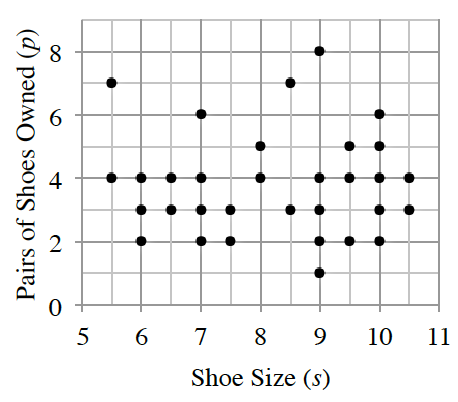### Home > CCAA8 > Chapter 6 Unit 7 > Lesson CCA: 6.2.4 > Problem6-99

6-99.

Make a conjecture about what $r$ is for the following scatterplot. Make a conjecture about what the $LSRL$ equation might be. Homework Help ✎

Is there any linear association between the variables?

$r ≈ 0$; Answers will vary for $LSRL$, but the average number of pairs appears to be about $3.8$ which is an $LSRL$ of $y = 3.8$.CCA 6-99 Student eTool